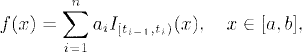# THE WIENER INTEGRAL

The Wiener integral is a simple stochastic integral where the integrand is a deterministic function. In this chapter, we present a definition and some results of the Wiener integral.

# 31.1 Basic Concepts and Facts

Definition 31.1 (Function Space L2[a, b]). The function spacedenotes the space of all real-valued square Lebesgue integrable functions on [a, b], where μ is the Lebesgue measure.

Definition 31.2 (Function Space L2(Ω,, P)). The function spacedenotes the space of all square integrable real-valued random variables with inner productX, Y= E(XY).

Definition 31.3 (Wiener Integral of Step Functions). Let f be a step function in L2[a, b] given bywhere a = t0 < t1 < ··· < tn = b. The Wiener integral of f is defined as

(31.1)where {Bt}t≥0 is the standard ...

Get Measure, Probability, and Mathematical Finance: A Problem-Oriented Approach now with the O’Reilly learning platform.

O’Reilly members experience live online training, plus books, videos, and digital content from nearly 200 publishers.